# Background

Let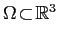be a bounded, simply or multiply connected, domain in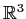with Lipschitz boundary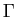occupying a homogeneous isotropic elastic solid that is characterized by the Lamé parameters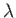and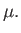In linear elasticity, the Lamé equation which describes the static equilibrium of a deformable body in terms of the displacement vector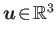is defined as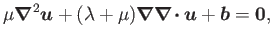where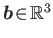represents a body force prescribed on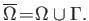To deal with the Lamé equation, let where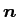be the unit normal todirected towards the exterior of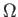and let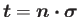be the traction vector onassociated with the displacement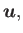where the stress tensor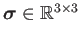is introduced as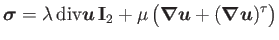with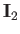denoting the symmetric second-order identity tensor onand the superscript "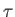" representing the transpose symbol. A solutionof the Lamé equation admits an integral representation, known as the Somigliana identity, expressed as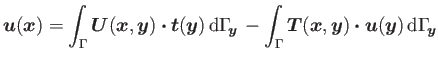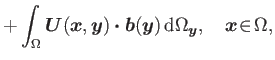where the kernels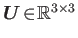and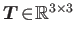are given respectively as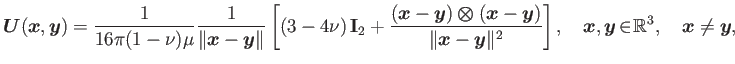and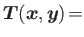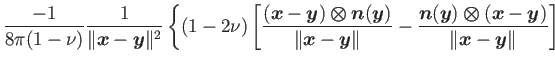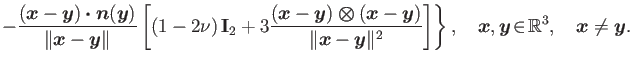Here the elastic constant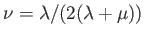is called the Poisson ratio of the solidand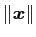is the usual Euclidean norm indefined as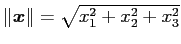. Moreover, it was established in  that the Newton potential admits a boundary representation as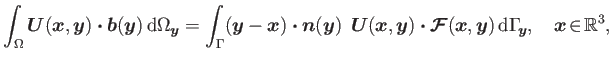where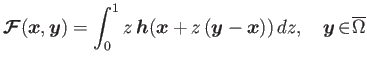with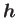denoting an extension of the body force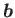into any ball centered  at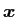and containing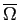. In particular, a continuationof the body forcecan be specified as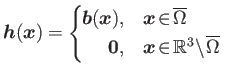This representation of the elastic Newton potential in term of surface integral allows a numerical solution of the Lamé equation that does not require a volume-fitted mesh.

# Solution via a boundary element method

To approximately solve the Lamé equation via a Boundary Element Method (BEM), the surfaceis usually discretized into flat triangles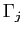using a mesh generation software (e.g. CUBIT).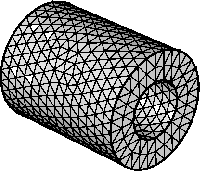With reference to , the vector fieldsandare assumed to have a polynomial variation over each triangle (boundary element)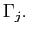Galerkin BEM

# References

 S. Nintcheu Fata.
Explicit expressions for three-dimensional boundary integrals in linear elasticity.
J. Comput. Appl. Math., 235(15):4480-4495, 2011.
 S. Nintcheu Fata.
Boundary integral approximation of volume potentials in three-dimensional linear elasticity.
J. Comput. Appl. Math., 242(1):275-284, 2013.